# CSE 421 Algorithms Richard Anderson Lecture 16 Dynamic

• Slides: 42
Download presentation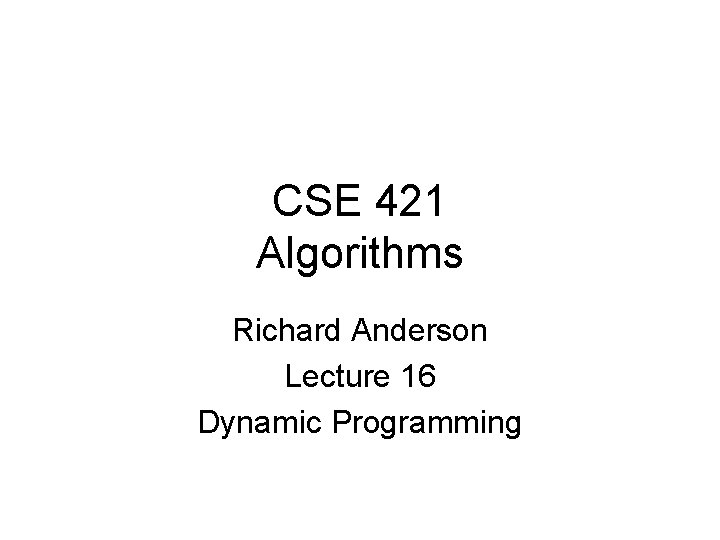CSE 421 Algorithms Richard Anderson Lecture 16 Dynamic ProgrammingDynamic Programming • Weighted Interval Scheduling • Given a collection of intervals I 1, …, In with weights w 1, …, wn, choose a maximum weight set of non-overlapping intervals 4 6 3 5 7 6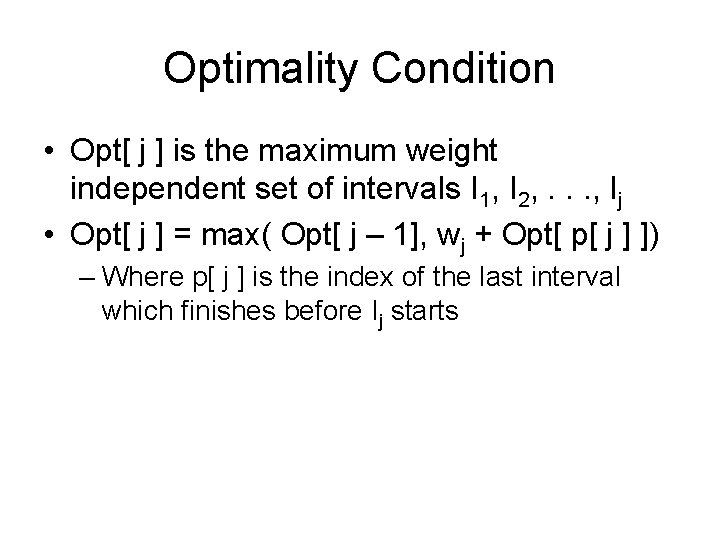Optimality Condition • Opt[ j ] is the maximum weight independent set of intervals I 1, I 2, . . . , Ij • Opt[ j ] = max( Opt[ j – 1], wj + Opt[ p[ j ] ]) – Where p[ j ] is the index of the last interval which finishes before Ij startsAlgorithm Max. Value(j) = if j = 0 return 0 else return max( Max. Value(j-1), wj + Max. Value(p[ j ])) Worst case run time: 2 n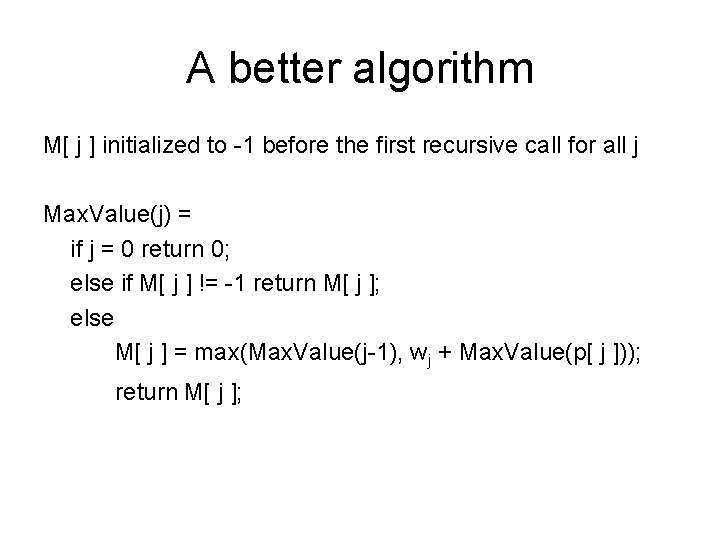A better algorithm M[ j ] initialized to -1 before the first recursive call for all j Max. Value(j) = if j = 0 return 0; else if M[ j ] != -1 return M[ j ]; else M[ j ] = max(Max. Value(j-1), wj + Max. Value(p[ j ])); return M[ j ];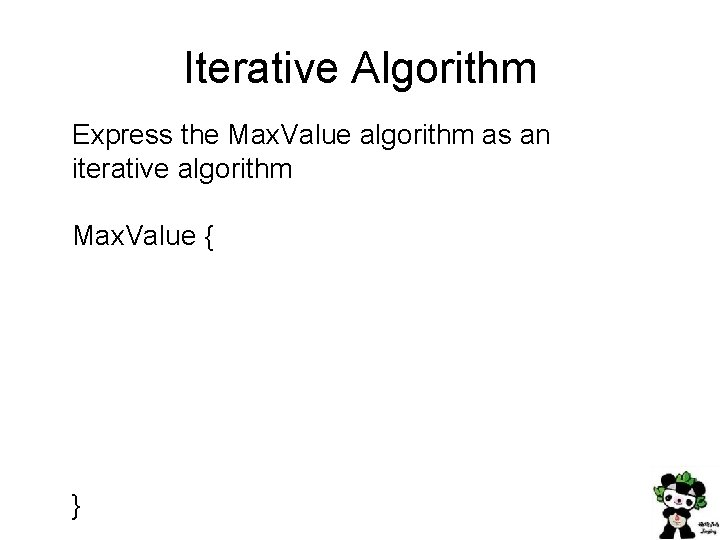Iterative Algorithm Express the Max. Value algorithm as an iterative algorithm Max. Value { }Fill in the array with the Opt values Opt[ j ] = max (Opt[ j – 1], wj + Opt[ p[ j ] ]) 2 4 7 4 6 7 6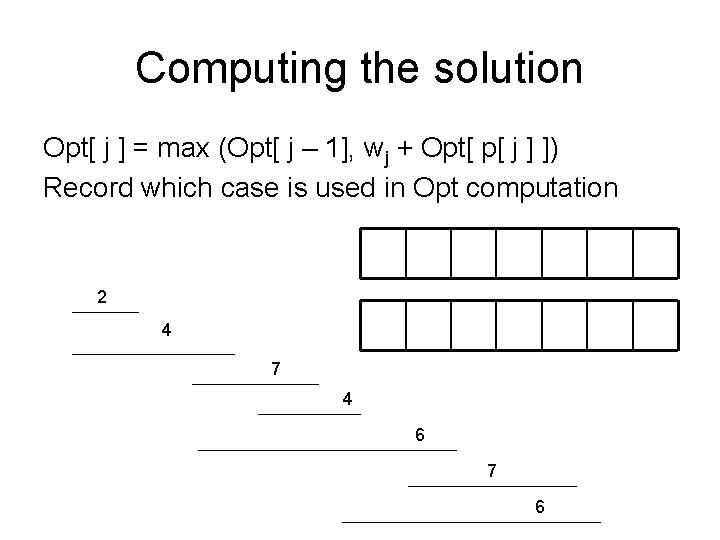Computing the solution Opt[ j ] = max (Opt[ j – 1], wj + Opt[ p[ j ] ]) Record which case is used in Opt computation 2 4 7 4 6 7 6Dynamic Programming • The most important algorithmic technique covered in CSE 421 • Key ideas – Express solution in terms of a polynomial number of sub problems – Order sub problems to avoid recomputation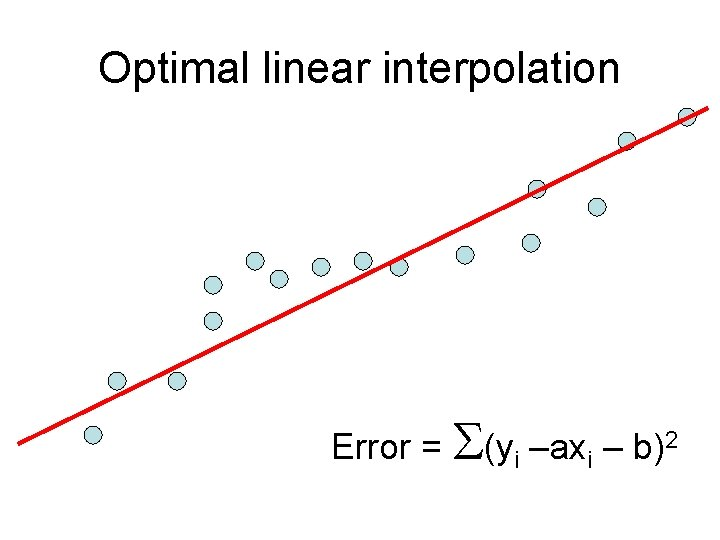Optimal linear interpolation Error = S(yi –axi – b)2Determine set of K lines to minimize errorOptk[ j ] : Minimum error approximating p 1…pj with k segments Express Optk[ j ] in terms of Optk-1, …, Optk-1[ j ] Optk[ j ] = mini {Optk-1[ i ] + Ei, j}Optimal sub-solution property Optimal solution with k segments extends an optimal solution of k-1 segments on a smaller problem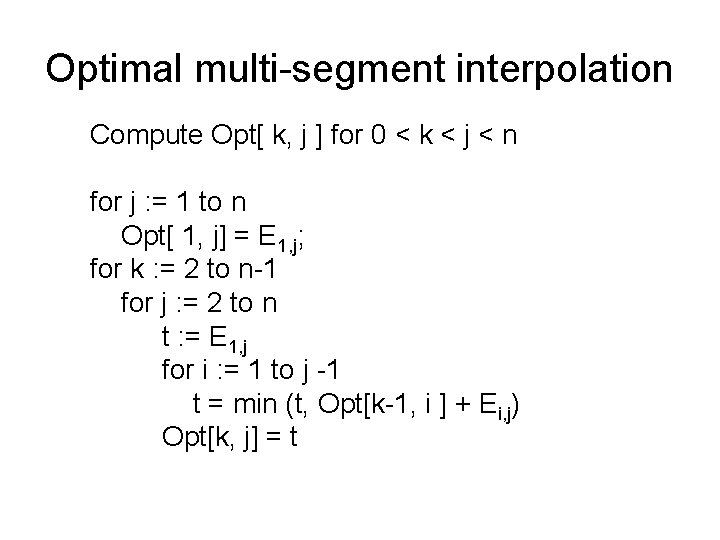Optimal multi-segment interpolation Compute Opt[ k, j ] for 0 < k < j < n for j : = 1 to n Opt[ 1, j] = E 1, j; for k : = 2 to n-1 for j : = 2 to n t : = E 1, j for i : = 1 to j -1 t = min (t, Opt[k-1, i ] + Ei, j) Opt[k, j] = tDetermining the solution • When Opt[ k , j ] is computed, record the value of i that minimized the sum • Store this value in a auxiliary array • Use to reconstruct solution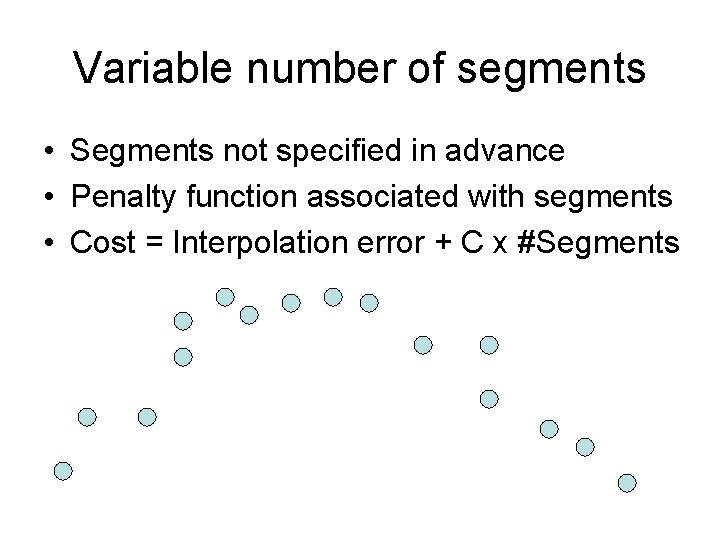Variable number of segments • Segments not specified in advance • Penalty function associated with segments • Cost = Interpolation error + C x #Segments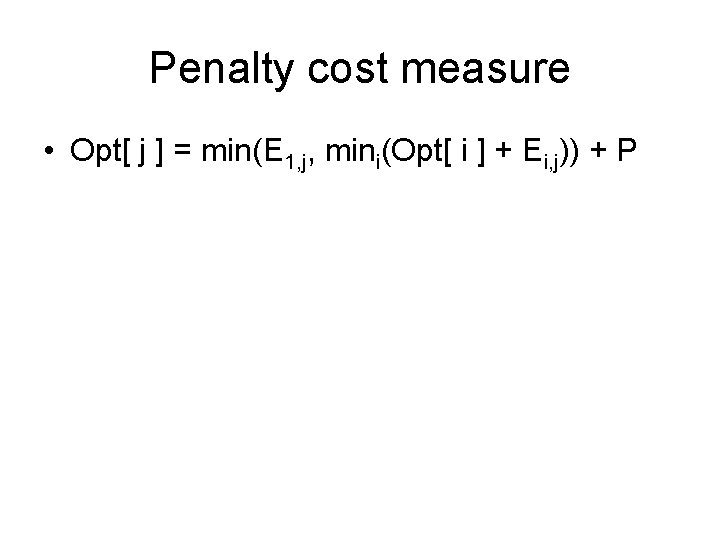Penalty cost measure • Opt[ j ] = min(E 1, j, mini(Opt[ i ] + Ei, j)) + PSubset Sum Problem • Let w 1, …, wn = {6, 8, 9, 11, 13, 16, 18, 24} • Find a subset that has as large a sum as possible, without exceeding 50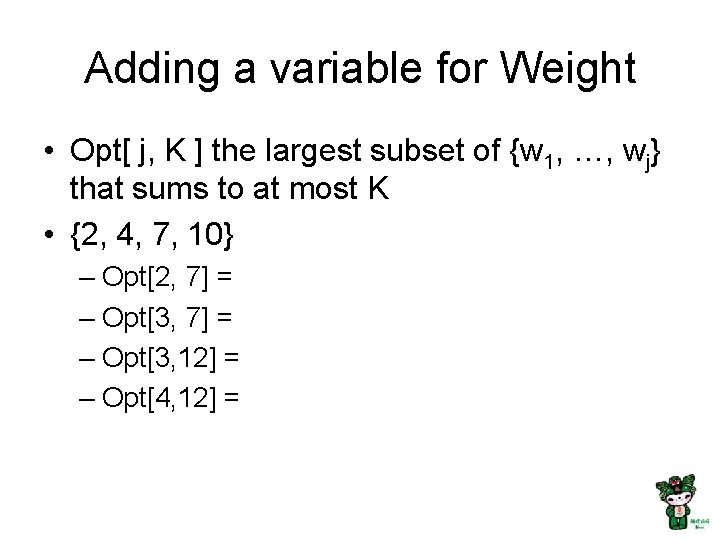Adding a variable for Weight • Opt[ j, K ] the largest subset of {w 1, …, wj} that sums to at most K • {2, 4, 7, 10} – Opt[2, 7] = – Opt[3, 12] = – Opt[4, 12] =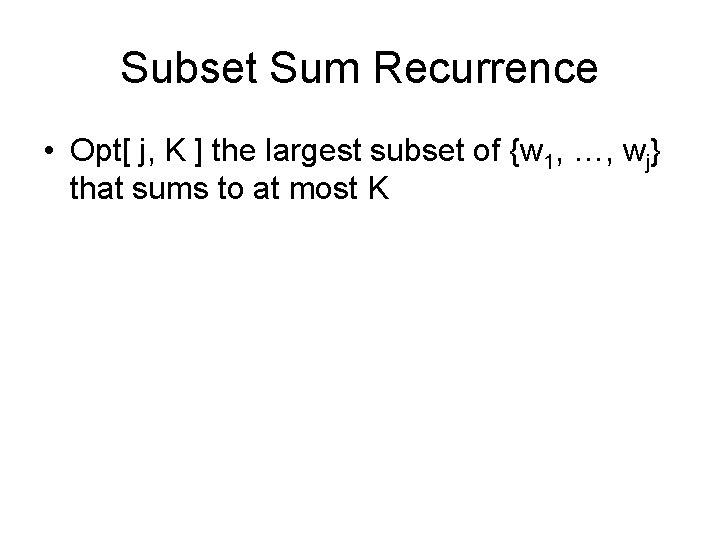Subset Sum Recurrence • Opt[ j, K ] the largest subset of {w 1, …, wj} that sums to at most K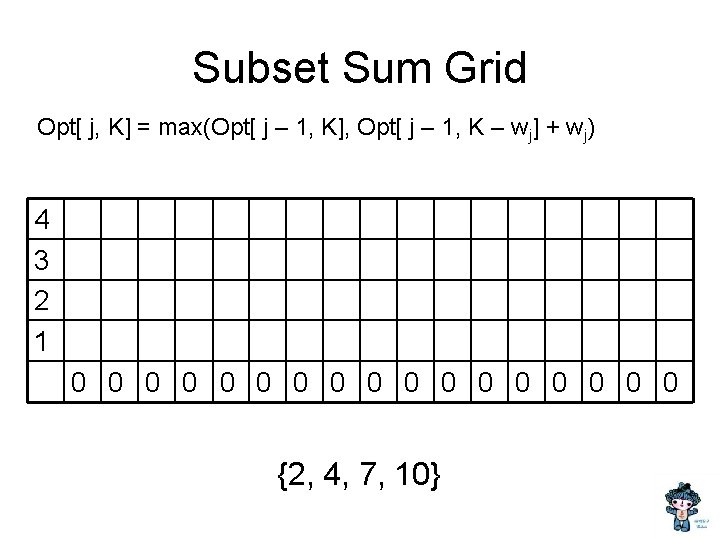Subset Sum Grid Opt[ j, K] = max(Opt[ j – 1, K], Opt[ j – 1, K – w j] + wj) 4 3 2 1 0 0 0 0 0 {2, 4, 7, 10}Subset Sum Code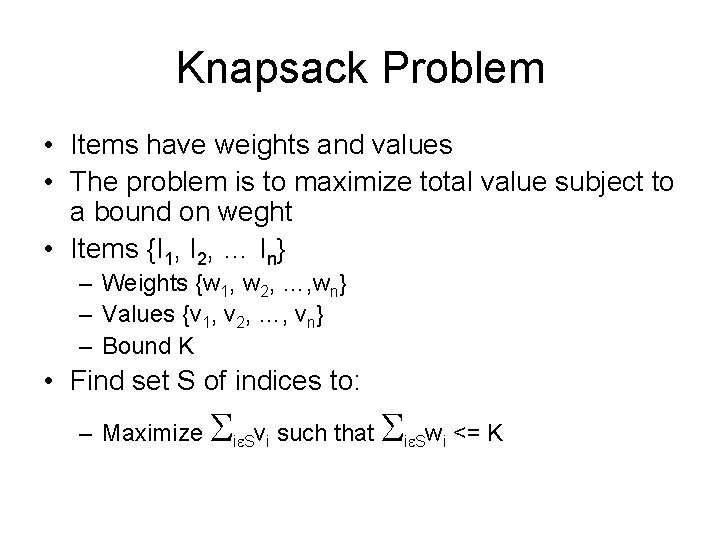Knapsack Problem • Items have weights and values • The problem is to maximize total value subject to a bound on weght • Items {I 1, I 2, … In} – Weights {w 1, w 2, …, wn} – Values {v 1, v 2, …, vn} – Bound K • Find set S of indices to: – Maximize S ie. Svi such that S ie. Swi <= KKnapsack Recurrence Subset Sum Recurrence: Opt[ j, K] = max(Opt[ j – 1, K], Opt[ j – 1, K – w j] + wj) Knapsack Recurrence:Knapsack Grid Opt[ j, K] = max(Opt[ j – 1, K], Opt[ j – 1, K – w j] + vj) 4 3 2 1 0 0 0 0 0 Weights {2, 4, 7, 10} Values: {3, 5, 9, 16}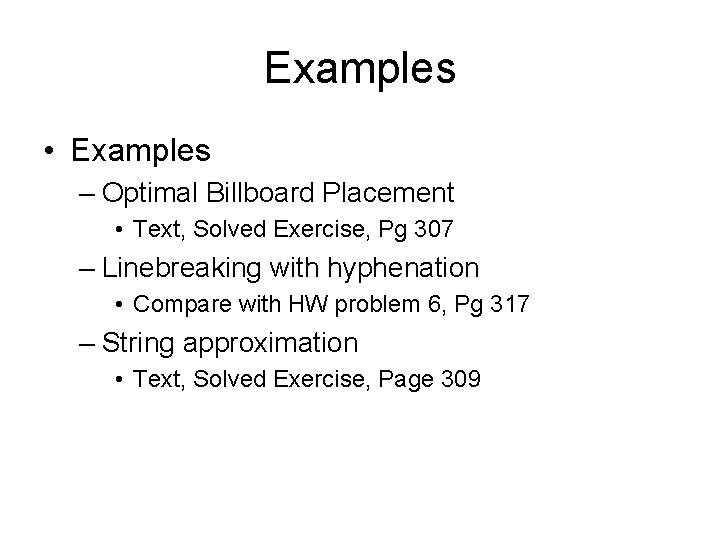Examples • Examples – Optimal Billboard Placement • Text, Solved Exercise, Pg 307 – Linebreaking with hyphenation • Compare with HW problem 6, Pg 317 – String approximation • Text, Solved Exercise, Page 309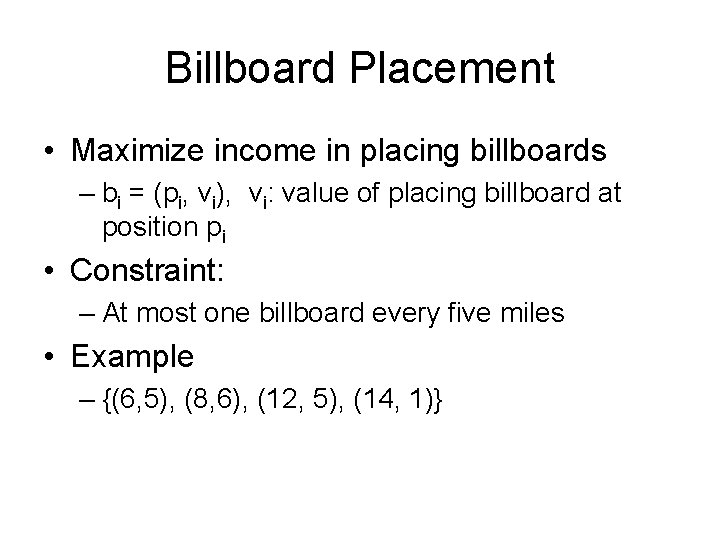Billboard Placement • Maximize income in placing billboards – bi = (pi, vi), vi: value of placing billboard at position pi • Constraint: – At most one billboard every five miles • Example – {(6, 5), (8, 6), (12, 5), (14, 1)}Design a Dynamic Programming Algorithm for Billboard Placement • Compute Opt, Opt, . . . , Opt[n] • What is Opt[k]? Input b 1, …, bn, where bi = (pi, vi), position and value of billboard iOpt[k] = fun(Opt, …, Opt[k-1]) • How is the solution determined from sub problems? Input b 1, …, bn, where bi = (pi, vi), position and value of billboard iSolution j = 0; // j is five miles behind the current position // the last valid location for a billboard, if one placed at P[k] for k : = 1 to n while (P[ j ] < P[ k ] – 5) j : = j + 1; j : = j – 1; Opt[ k] = Max(Opt[ k-1] , V[ k ] + Opt[ j ]);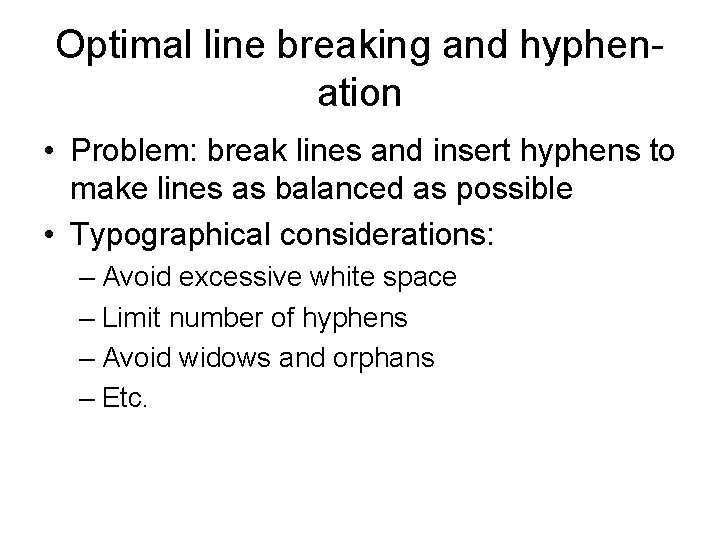Optimal line breaking and hyphenation • Problem: break lines and insert hyphens to make lines as balanced as possible • Typographical considerations: – Avoid excessive white space – Limit number of hyphens – Avoid widows and orphans – Etc.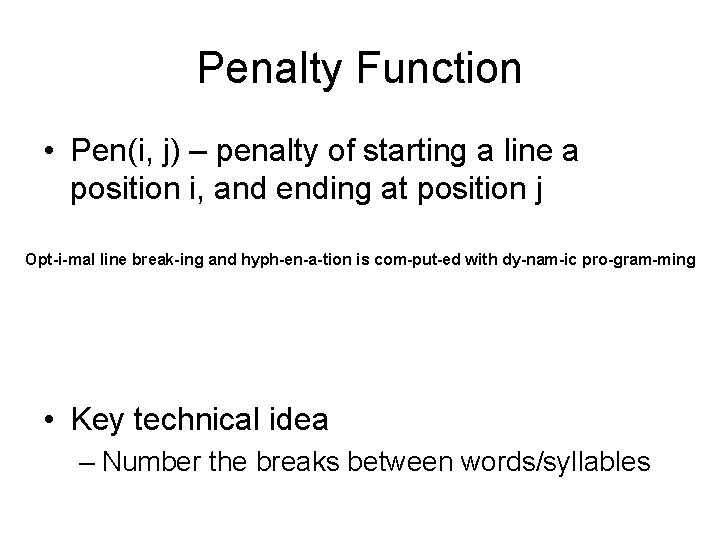Penalty Function • Pen(i, j) – penalty of starting a line a position i, and ending at position j Opt-i-mal line break-ing and hyph-en-a-tion is com-put-ed with dy-nam-ic pro-gram-ming • Key technical idea – Number the breaks between words/syllablesDesign a Dynamic Programming Algorithm for Optimal Line Breaking • Compute Opt, Opt, . . . , Opt[n] • What is Opt[k]?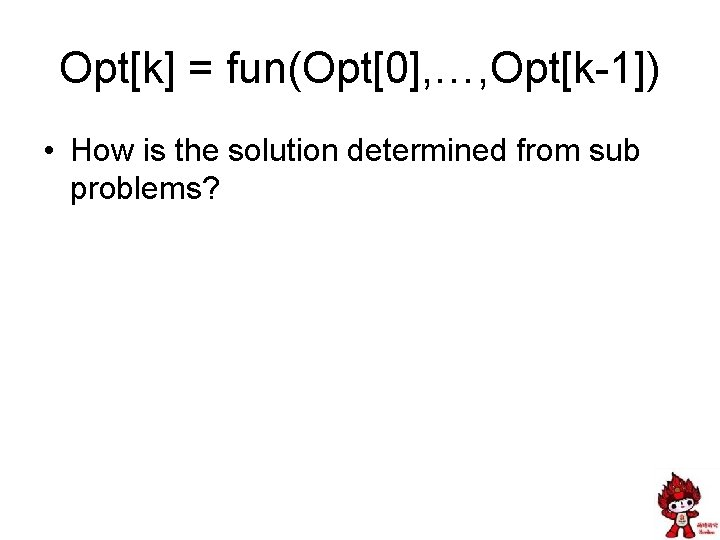Opt[k] = fun(Opt, …, Opt[k-1]) • How is the solution determined from sub problems?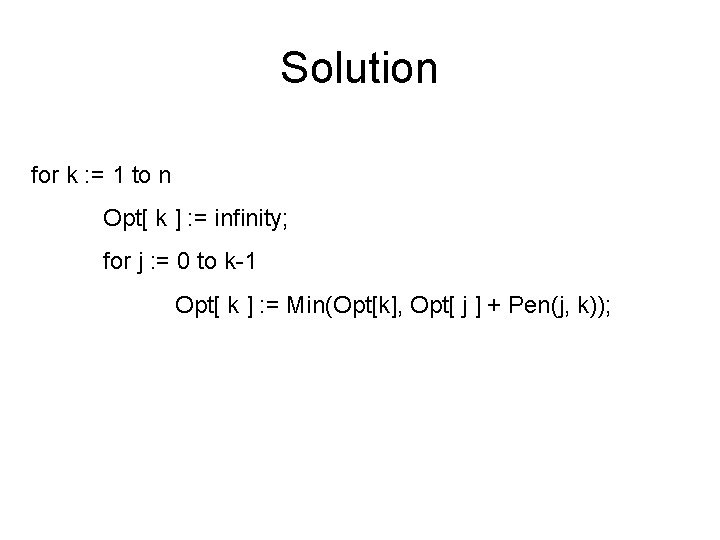Solution for k : = 1 to n Opt[ k ] : = infinity; for j : = 0 to k-1 Opt[ k ] : = Min(Opt[k], Opt[ j ] + Pen(j, k));But what if you want to layout the text? • And not just know the minimum penalty?Solution for k : = 1 to n Opt[ k ] : = infinity; for j : = 0 to k-1 temp : = Opt[ j ] + Pen(j, k); if (temp < Opt[ k ]) Opt[ k] = temp; Best[ k ] : = j;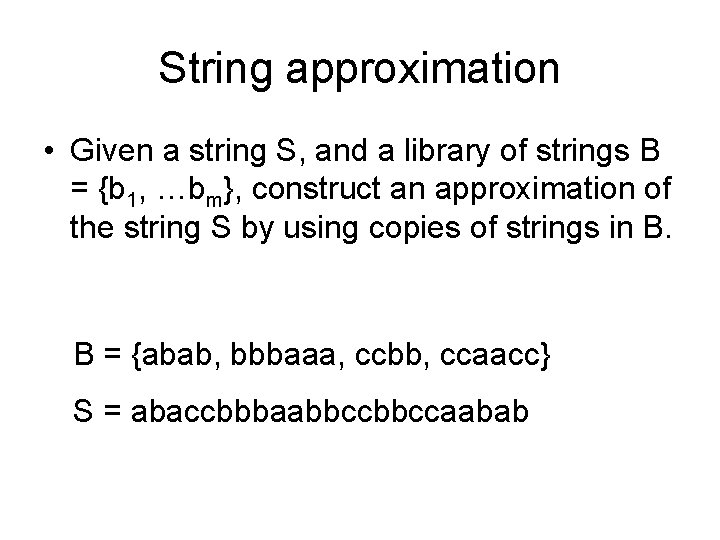String approximation • Given a string S, and a library of strings B = {b 1, …bm}, construct an approximation of the string S by using copies of strings in B. B = {abab, bbbaaa, ccbb, ccaacc} S = abaccbbbaabbccaababFormal Model • Strings from B assigned to nonoverlapping positions of S • Strings from B may be used multiple times • Cost of d for unmatched character in S • Cost of g for mismatched character in S – Mis. Match(i, j) – number of mismatched characters of bj, when aligned starting with position i in s.Design a Dynamic Programming Algorithm for String Approximation • Compute Opt, Opt, . . . , Opt[n] • What is Opt[k]? Target string S = s 1 s 2…sn Library of strings B = {b 1, …, bm} Mis. Match(i, j) = number of mismatched characters with bj when aligned starting at position i of S.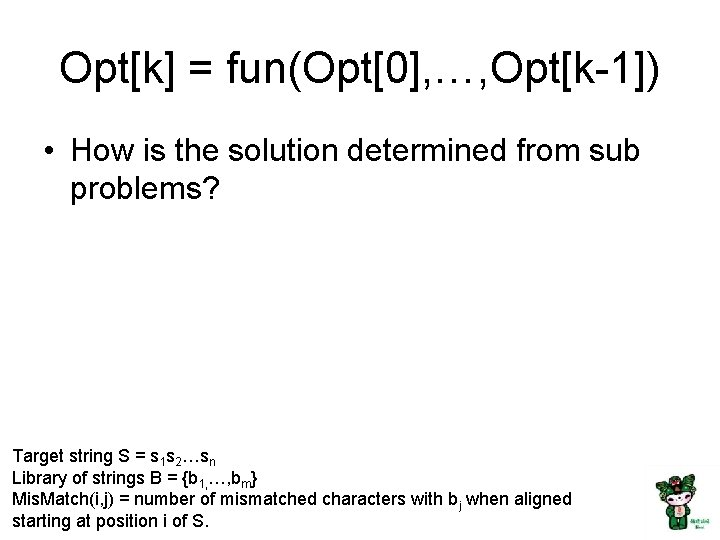Opt[k] = fun(Opt, …, Opt[k-1]) • How is the solution determined from sub problems? Target string S = s 1 s 2…sn Library of strings B = {b 1, …, bm} Mis. Match(i, j) = number of mismatched characters with bj when aligned starting at position i of S.Solution for i : = 1 to n Opt[k] = Opt[k-1] + d; for j : = 1 to |B| p = i – len(bj); Opt[k] = min(Opt[k], Opt[p-1] + g Mis. Match(p, j));